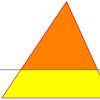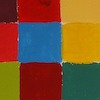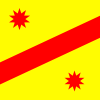# Resources tagged with: Constructions

Filter by: Content type:
Age range:
Challenge level:

### There are 22 results

Broad Topics > Transformations and constructions > Constructions### A Rational Search

##### Age 16 to 18Challenge Level

Investigate constructible images which contain rational areas.### Kissing

##### Age 16 to 18Challenge Level

Two perpendicular lines are tangential to two identical circles that touch. What is the largest circle that can be placed in between the two lines and the two circles and how would you construct it?### Circle Scaling

##### Age 14 to 16Challenge Level

Describe how to construct three circles which have areas in the ratio 1:2:3.### Curvy Areas

##### Age 14 to 16Challenge Level

Have a go at creating these images based on circles. What do you notice about the areas of the different sections?### Golden Construction

##### Age 16 to 18Challenge Level

Draw a square and an arc of a circle and construct the Golden rectangle. Find the value of the Golden Ratio.### Gold Again

##### Age 16 to 18Challenge Level

Without using a calculator, computer or tables find the exact values of cos36cos72 and also cos36 - cos72.### LOGO Challenge 8 - Rhombi

##### Age 7 to 16Challenge Level

Explore patterns based on a rhombus. How can you enlarge the pattern - or explode it?### Moving Squares

##### Age 14 to 16Challenge Level

How can you represent the curvature of a cylinder on a flat piece of paper?### The Medieval Octagon

##### Age 14 to 16Challenge Level

Medieval stonemasons used a method to construct octagons using ruler and compasses... Is the octagon regular? Proof please.### LOGO Challenge 2 - Diamonds Are Forever

##### Age 7 to 16Challenge Level

The challenge is to produce elegant solutions. Elegance here implies simplicity. The focus is on rhombi, in particular those formed by jointing two equilateral triangles along an edge.### Triangle Midpoints

##### Age 14 to 16Challenge Level

You are only given the three midpoints of the sides of a triangle. How can you construct the original triangle?### Pentabuild

##### Age 16 to 18Challenge Level

Explain how to construct a regular pentagon accurately using a straight edge and compass.### Half a Triangle

##### Age 14 to 16Challenge Level

Construct a line parallel to one side of a triangle so that the triangle is divided into two equal areas.### Pareq Exists

##### Age 14 to 16Challenge Level

Prove that, given any three parallel lines, an equilateral triangle always exists with one vertex on each of the three lines.### The Square Under the Hypotenuse

##### Age 14 to 16Challenge Level

Can you work out the side length of a square that just touches the hypotenuse of a right angled triangle?### Folding Squares

##### Age 14 to 16Challenge Level

The diagonal of a square intersects the line joining one of the unused corners to the midpoint of the opposite side. What do you notice about the line segments produced?### Two Points Plus One Line

##### Age 14 to 16Challenge Level

Draw a line (considered endless in both directions), put a point somewhere on each side of the line. Label these points A and B. Use a geometric construction to locate a point, P, on the line,. . . .### Three Tears

##### Age 14 to 16Challenge Level

Construct this design using only compasses### Folding Fractions

##### Age 14 to 16Challenge Level

What fractions can you divide the diagonal of a square into by simple folding?### Squirty

##### Age 14 to 16Challenge Level

Using a ruler, pencil and compasses only, it is possible to construct a square inside any triangle so that all four vertices touch the sides of the triangle.### Cool as Ice

##### Age 11 to 16Challenge Level

Design and construct a prototype intercooler which will satisfy agreed quality control constraints.### Close to Triangular

##### Age 14 to 16Challenge Level

Drawing a triangle is not always as easy as you might think!